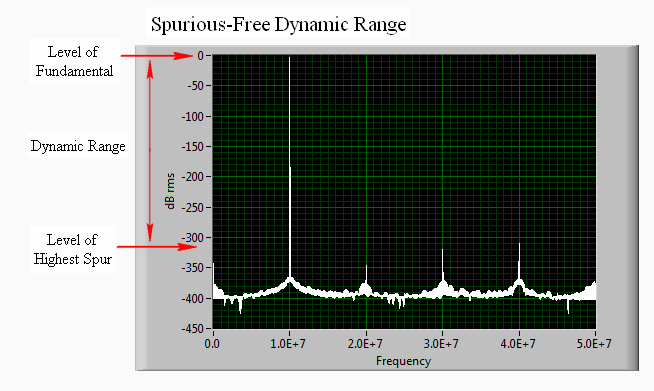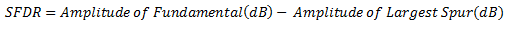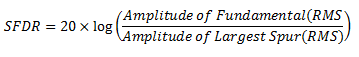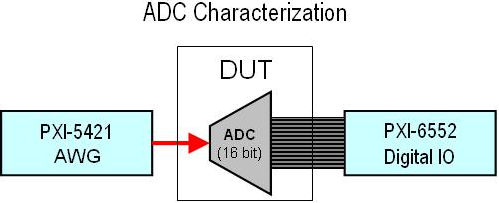# Specifications Explained: Spurious-Free Dynamic Range (SFDR)

Publish Date: Feb 06, 2018 | 4 Ratings | 3.75 out of 5 | Print

## Overview

This article explains and defines the Spurious-Free Dynamic Range (SFDR) specification used in some NI devices and modules like NI Dynamic Signal Acquisition (DSA).

### 1. Introduction

Spurious-Free Dynamic Range (SFDR) is a crucial specification that you can use to characterize the dynamic performance of a signal generator. SFDR specifies the relationship between the amplitude of the fundamental frequency being generated and the amplitude of the most prominent harmonic. In an ideal world, the frequency domain of a pure analog signal has all power concentrated at the desired frequency. However, due to noise and the nonlinearity of components, even the best signal generators also generate frequency content at harmonics (or multiples) of the desired tone.

For example, when generating a 10 MHz sine wave, you can observe harmonics at 20 MHz, 30 MHz, and so on.  These harmonics are also referred to as spurs.

The dynamic range between the fundamental tone and the largest spur is called spurious-free dynamic range (SFDR). SFDR is the measure of the ratio between the fundamental signal and the largest harmonically or nonharmonically related spur from DC to half of the sampling rate. You can calculate this specification visually by observing the following graph.To measure the SFDR of a signal generator, a tone is generated at a given frequency. A spectrum analyzer is then used to measure the amplitude of the fundamental tone and the amplitude of the next highest tone. Typically, this is one of the harmonics.

Using decibels, it is easy to calculate SFDR:On the graph above, the second harmonic is the second highest tone and the SFDR is approximately 310 dBc. As a matter of perspective, let us say the SFDR of a signal is 70 dBc. This means that the amplitude of spurious signals is always less than 0.0003162 times the amplitude of the fundamental frequency.

Given RMS voltages, you can calculate SFDR with the following equation:For many applications requiring a clean sinusoid, it is important that a signal generator has a good SFDR performance. For example, consider analog-to-digital-converter (ADC) characterization below.For ADC characterization, it is important to measure the harmonic imperfections of the ADC. Thus, it is crucial that the stimulus to the device under test is as spectrally pure as possible.

### Ratings

Rate this document